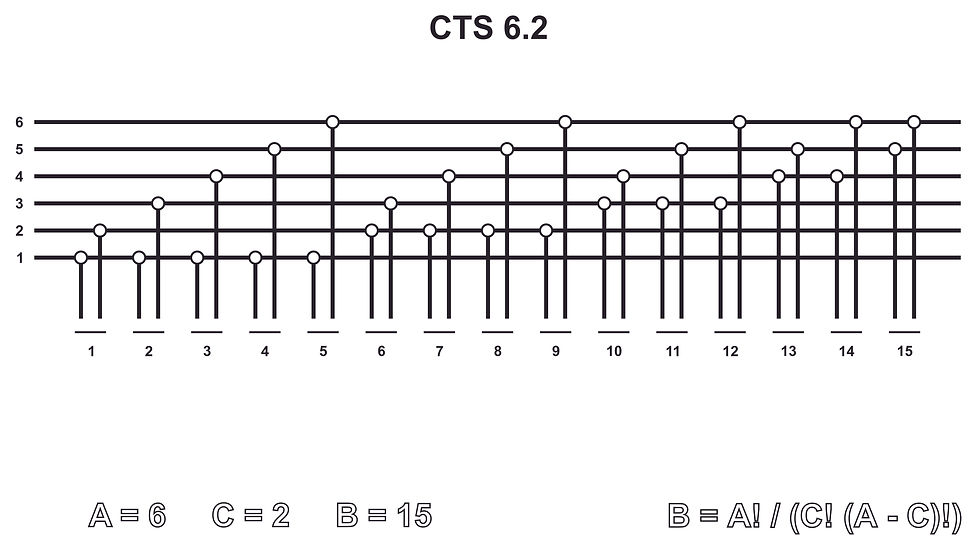top of page

To begin with, we will use a "Pascal's triangle" (also known under other names)

that we will divide into A and B and on the left side of that add a table C.

Now, a series of numbers from 0 to infinitely, we will call A.

Everything else below A we will call B.

In Table A red circle marks number 4, which means we have 4 lines or 4 addresses on side A.In the Table C, the red circle indicates the number 3, which means that we will combine lines or addresses of the side A and make groups of them, wich will contain 3 of 4 lines or adresses

in one group.

The combined groups schould never repeate.

Combinations of lines or addresses of the side A will be shown as a group of vertical lines and the joint points.

Figure below shows the first of several possible combinations of A=4, C=3.The number 4 in the Table B is marked with a red circle because it is a place where the values

of the table A (vertically) and table C (horizontally) intersect or meet.

The number 4 in table B means that on the side B we have 4 adresses without repeating them.

Also, you can notice that at A=4 and C=2, we would have B=6, or 6 adresses on the B side,

or at the A=4 and C=4, we would have B=1, or just 1 adress on B side.

It is interesting to note that when C ≈ A/2, then B = max, or B is at maximum.The following example shows CTS when A = 6, C = 2 and B = 15.The next example shows CTS when A = 6, C = 3 and B = 20.bottom of page